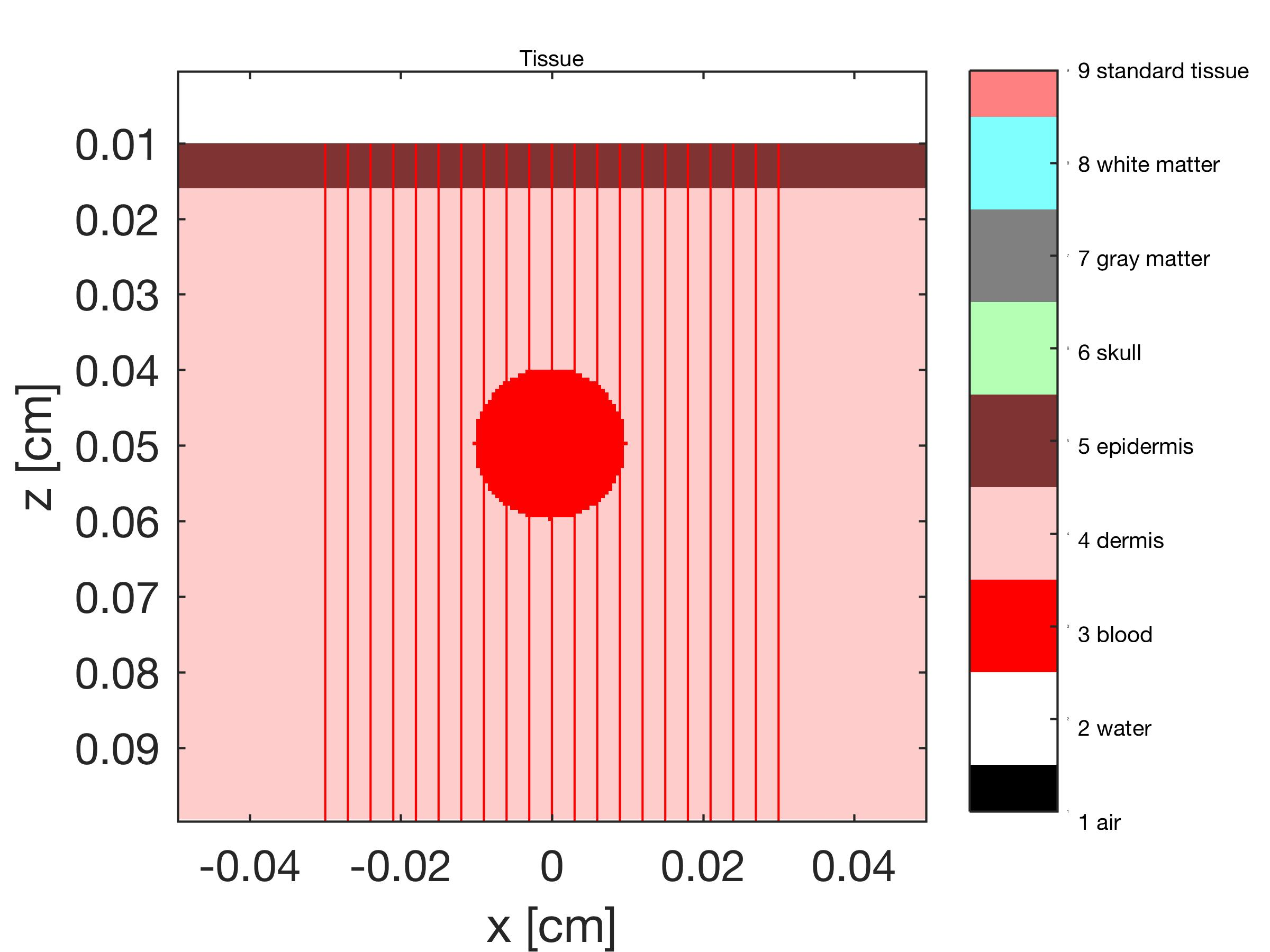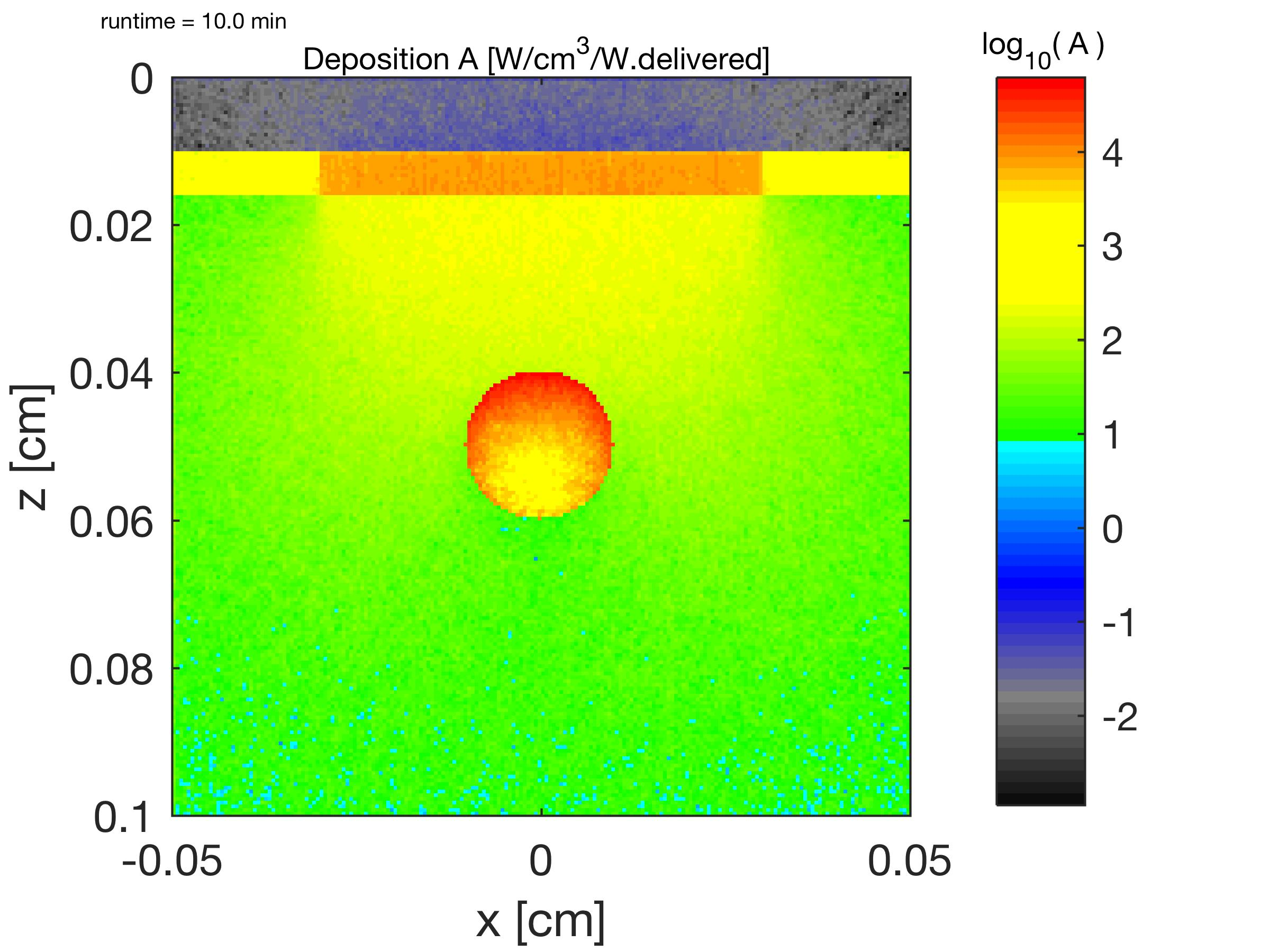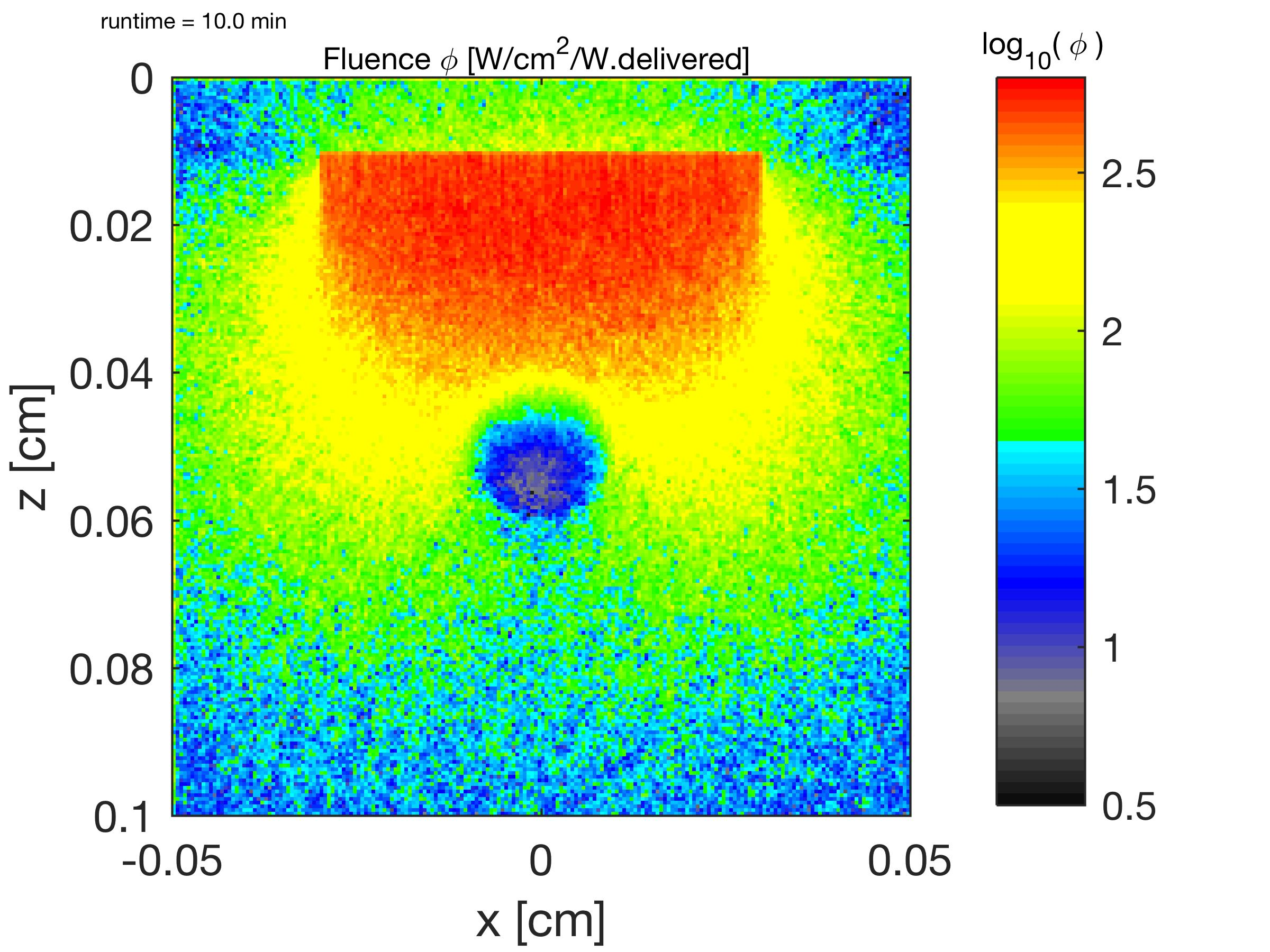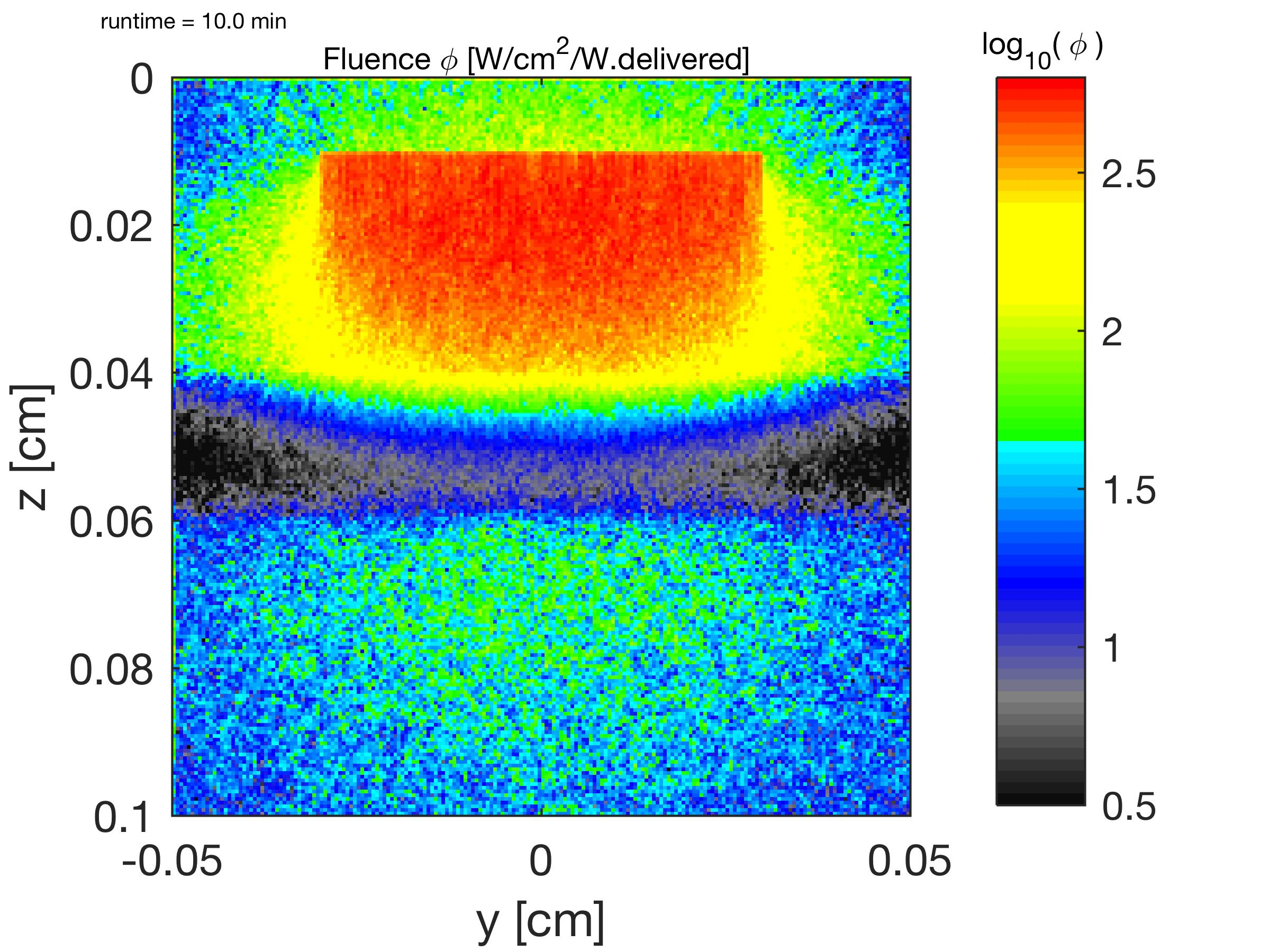# mcxyz.c

Version July 22, 2019.

You can download a .zip file holding mcxyz.c and support programs written in MATLAB:

1. mcxyz_22july2019.zip. This .zip file is 28 MB in size because it contains two big files (the input file skinvessel_T.bin (8MB), and the output file skinvessel_F.bin (32MB) (see updates in notes_update.txt). .

2. mcxyz_22july2019minimal.zip. This .zip file is 1.5 MB in size because it it does not contains the two big files. You create skinvessel_T.bin using maketissue.m. Running mcxyz.c creates skinvessel_F.bin.

Brief instructions:
1. In MATLAB, run maketissue.m, which will create skinvessel_T.bin.
2. Compile mcxyz.c: cc -o mcxyz mcxyz.c (on UNIX), or using the compiler for your platform.
3. Run mcxyz.c: mcxyz skinvessel . This will load the skinvessel_T.bin and skinvessel_H.mci needed by mcxyz.c, and run it.
4. In MATLAB, run lookmcxyz.m, which will read skinvessel_T.bin and skinvessel_F.bin and draw figures.

I would appreciate any feedback.

Version June 1, 2017.
Thanks to Anh Phong Tran for feedback
Version Feb. 8, 2017.
Version Oct. 10, 2014.
Thanks to Angelina (Angelina Ryzhov) and Baki (Reheman Baikejiang) for feedback.
Version March 4, 2013.
Thanks to Marleen Keijzer, Scott Prahl, Lihong Wang and Ting Li, who have all contributed to the evolution of mcxyz.c.

## Overview

The program mcxyz.c is a computer simulation of the distribution of light within a complex tissue that consists of many different types of tissues, each with its own optical properties. The program uses the Monte Carlo method of sampling probabilities for the stepsize of photon movement between scattering events and for the angles (θ,ψ) of photon scattering.

All boundary conditions are matched. Does not yet handle mismatched boundaries.

mcxyz.c is written in ANSI standard C, and is easily compiled by any standard compiler. It reads input files (myname_T.bin, myname_H.mci) created by a MATLAB program maketissue.m, using a standard format. Therefore, other programs can also prepare the input files.

The 3D Monte Carlo generates an output file of relative fluence rate, F(y,x,z) [W/cm2 per W delivered] or [1/cm2]. The spatial distribution of absorption is obtained by the product of the fluence rate and the absorption coefficient: A(y,x,z) [1/cm3] = F(y,x,z) x muav(T(y,x,z), where muav(i) [cm-1] is the absorption coefficient of the ith tissue type (the v is for voxel).

## Figure 1

An example showing the results of a simulation that includes air, epidermis, dermis, and a blood vessel. The tissue list also includes skull, gray matter and white matter (brain) but these tissue types are not used in this example. Green 532-nm light is delivered to the air/epidermis surface as a uniform-field 0.6-cm-diameter circular collimated beam. A 0.1-cm-diameter blood vessel is located at x,z = 0,0.0500 cm, and runs along the y axis. In this simulation, there are 200 bins in x,y,z, i.e., T(200,200,200) is used to generate F(200,200,200). The program was run for 10 min, launching 825,219 photons.

### Figure 1A

The tissue types T(z,x)@y are shown. The red lines show the photon paths if there were no scattering or absorption. The blood vessel extends along the y axis, and its cross-section is seen.### Figure 1B

The relative energy deposition A(z,x)@y [1/cm3 = W/cm3/W.delivered] is shown. The light delivered through the water is not shown; light is delivered to tissue surface as z = 0.0100. Deposition in water above skin is due to escaping light only. ( log10(A [cm-3]) )### Figure 1C

The relative fluence rate φ(z,x)@y [1/cm2] is shown. The fluence rate of light escaping out of the skin at the epidermal surface into the air is also shown. ( log10(φ [cm-2]) )/p>

### Figure 1D

The relative fluence rate φ(z,u)@x [1/cm2] is shown, where x equals the middle of the blood vessel, which appears as the horizontal bar of black at z = 0.4 cm due to strong absorption by blood. ( log10(φ [cm-2]) ).## In the following sections, you will learn

1. how to use makeTissueList.m (MATLAB) to create a library of tissue optical properties
2. how to use maketissue.m (MATLAB) to create the inputfiles (myname_T.bin, myname_H.mci) for use by mcxyz.c
3. how to use mcxyz.c
4. how to use lookmcxyz.m (MATLAB) to look at the results.

You can download a .zip file containing the 4 programs listed above, and supporting files, and the example simulation of Figure 1: mcxyz_22july2019.zip. The file contains:

 3372 bytes makeTissueList.m 7712 bytes maketissue.m 33925 bytes mcxyz.c 6035 bytes lookmcxyz.m and supporting files... 32000000 bytes skinvessel_F.bin 8000000 bytes skinvessel_T.bin 342 bytes skinvessel_H.mci 494 bytes skinvessel_props.m 28191 bytes spectralLIB.mat 1261 bytes makec2f.m 815 bytes makecmap.m 931 bytes reportHmci.m 1125 bytes savepic.m 259847 bytes skinvessel_tissue.jpg 310731 bytes skinvessel_Azx.jpg 540763 bytes skinvessel_Fzx.jpg 600543 bytes skinvessel_Fzy.jpg

Finally, some example simulations will be presented to illustrate how to adjust simulations.

## How to use makeTissueList.m to create a library of tissue optical properties

To create a library of tissue types, with the associated tissue optical properties, the file spectralLIB.mat is used. This file contains the optical absorption properties, μa [cm-1], of

• oxygenated whole blood (150 g hemoglobin/liter or 2.33x10-3 M)
• deoxygenated whole blood
• water
• interior of typical cutaneous melanosome
• fat
as functions of wavelength from 300 to 1000 nm. You can download spectralLIB.mat with the mcxyz.zip file. You can view a text version of the file here: spectralLIB.dat.

To create the scattering properties, a generic function is used:

μs' = μs.500nm' (fRayleigh(λ/500nm)-4 + fMie(λ/500nm)-bMie)

where

• μs.500nm' = reduced scattering coeff. at 500 nm
• fRayleigh = fraction of Rayleigh scattering at 500 nm
• fMie = fraction of Mie scattering at 500 nm
• λ = wavelength [nm]
• bMie = scattering power of Mie scattering

Each tissue type is specified as follows, using the 4th tissue type, dermis, as an example:

```
j = 4;
tissue(j).name = 'dermis';
B       = 0.002;    % blood volume fraction
S       = 0.67;     % oxygen saturation of hemoglobin
W       = 0.65;     % water volume fraction
F       = 0.02;     % fat volume fraction
M       = 0;        % volume fraction of melanosomes
musp500 = 42.4;     % reduced scattering coeff. at 500 nm
[cm-1]
fray    = 0.62;     % fraction of Rayleigh scattering at 500 nm
fmie    = 1-fray;   % fraction of Mie scattering at 500 nm
bmie    = 1.0;      % scatter power for mie scattering
X       = [B*S B*(1-S) W F M]';
% [oxyBlood deoxyBlood Water Fat Melansomes]'
musp    = musp500*(fray*(nm/500).^-4 + fmie*(nm/500).^-bmie);
%reduced scattering
gg      = 0.90;
tissue(j).mua = MU*X;       % absorption coefficient
tissue(j).mus = musp/(1-gg);    % scattering coefficient
tissue(j).g   = gg;     % anisotropy of scattering

```
The above calculates the absorption (tissue(j).mua), the scattering (tissue(j).mus) and the anisotropy of scattering (tissue(j).g)) at the wavelength of interest. By adjusting B,S,W,F and M, the absorption properties are specified. By adjusting musp500, fray, bmie, the scattering properties are specified.

The function call tissue = makeTissueList(nm), where nm specifies the wavelength of interest in [nm], will yield a data structure called tissue with the structure:

• tissue(j).name
• tissue(j).mua
• tissue(j).mus
• tissue(j).g
• tissue(j).nm
where j selects the jth tissue type in the library. The parameter tissue(j).nm is the same single wavelength for all tissues, but is included for convenient recall.

In the example makeTissueList(nm) presented here, 9 tissue types are specified:

1. air
2. water
3. blood
4. dermis
5. epidermis
6. fat or lipid
7. skull
8. gray matter (brain)
9. white matter (brain)

The USER can create a variety of makeTissueList.m files, each with a different assortment of tissue types.
CAUTION: The tissue parameters chosen for the above tissue types are approximations, which create approximate optical properties at the chosen wavelength. The USER is responsible for improving on the choices of tissue parameters, so as to get more accurate optical properties.

For both air and water, a moderate scattering coefficient is specified so that the photon steps through the tissue, but the value of g is set to 1.0 so there is not photon deflection. Also a very very low absorption coefficient is specified (eg., μa = 0.0001 cm-1 for air, or the absorption of water at the chosen wavelength). Hence, the photon will step through air or water, and deposit a very small amount of photon weight in the voxels, thereby specifying its pathlength spent in the air or water. But the energy lost in the air is negligible, so it does not significantly influence the distribution of light. The absorption in water depends on the chosen wavelength. At the end of the program (discussed in How to use mcxy.c, below), the fluence rate φ is calculated by dividing the deposited energy by the very small μa which recovers the φ in the air and water.

View listing of makeTissueList.m: makeTissueList.m

## How to use maketissue.m to create a tissue

The program maketissue.m uses the tissue library tissue created by makeTissueList.m. The program creates a complex tissue, according to the user's adjustments of the following parameters in the program:

```%%% USER CHOICES %%%%%%%% <-------- You must set these parameters ------
SAVEON      = 1;        % 1 = save myname_T.bin, myname_H.mci
% 0 = don't save. Just check the program.
%
myname      = 'skinvessel';% name for files: myname_T.bin, myname_H.mci

time_min    = 10;       % time duration of the simulation [min]
<----- run time -----
nm          = 532;      % desired wavelength of simulation
Nbins       = 200;      % # of bins in each dimension of cube
binsize     = 0.0005;   % size of each bin, eg. [cm] or [mm]

% Set Monte Carlo launch flags
mcflag      = 0;        % launch: 0 = uniform beam, 1 = Gaussian, 2 =
isotropic pt.
% 3 = rectangular beam (use xfocus,yfocus for
% x,y halfwidths)
launchflag  = 0;        % 0 = let mcxyz.c calculate launch trajectory
% 1 = manually set launch vector.
boundaryflag = 2;       % 0 = no boundaries, 1 = escape at boundaries
% 2 = escape at surface only. No x, y, bottom z
% boundaries
%
% Sets position of source
xs          = 0;        % x of source
ys          = 0;        % y of source
zs          = 0.0101;   % z of source

% Set position of focus, so mcxyz can calculate launch trajectory
xfocus      = 0;        % set x,position of focus
yfocus      = 0;        % set y,position of focus
zfocus      = inf;      % set z,position of focus (=inf for collimated
beam)

% only used if mcflag == 0 or 1 or 3 (not 2=isotropic pt.)
waist       = 0.0300;   % 1/e radius of beam at focus

% only used if launchflag == 1 (manually set launch trajectory):
ux0         = 0.7;      % trajectory projected onto x axis
uy0         = 0.4;      % trajectory projected onto y axis
uz0         = sqrt(1 - ux0^2 - uy0^2); % such that ux^2 + uy^2 + uz^2 = 1
%%%%%%%%%%%%%%%%%%%%%%%%%
```
The program then enters the section
```    %%%%%%%%%%
% Prepare Monte Carlo
%     %%%
```
which sets up the parameters for the Monte Carlo simulation. (View the original code.)

The user then creates the desired complex tissue in the next section:

```%%%%%%
% CREATE TISSUE STRUCTURE T(y,x,z)
%   Create T(y,x,z) by specifying a tissue type (an integer)
%   for each voxel in T.
% %
%   Note: one need not use every tissue type in the tissue list.
%   The tissue list is a library of possible tissue types.
%
T = double(zeros(Ny,Nx,Nz));

T = T + 4;      % fill background with skin (dermis)

zsurf = 0.0100;  % position of air/skin surface

for iz=1:Nz % for every depth z(iz)

% air
if iz<=round(zsurf/dz)
T(:,:,iz) = 2;
end

% epidermis (60 um thick)
if iz>round(zsurf/dz) & iz<=round((zsurf+0.0060)/dz)
T(:,:,iz) = 5;
end

% blood vessel @ xc, zc, radius, oriented along y axis
xc      = 0;            % [cm], center of blood vessel
zc      = Nz/2*dz;      % [cm], center of blood vessel
for ix=1:Nx
xd = x(ix) - xc;    % vessel, x distance from vessel center
zd = z(iz) - zc;    % vessel, z distance from vessel
center
r  = sqrt(xd^2 + zd^2); % r from vessel center
if (r<=vesselradius)        % if r is within vessel
T(:,ix,iz) = 3; % blood
end

end %ix

end % iz
```
In the above, the user fills the backround with dermis, specifies the position of the air/skin surface (zsurf), then steps through each z(iz) depth position and determines if in air or epidermis, assigning the appropriate integer pointer value to all x,y elements at the z(iz) depth in the tissue array T(iy,ix,iz). Also, the presence of the blood vessel at depth z(iz) for each of the x,y positions is tested, based on the vessel radius being a set value (vesselradius).

When ready to save the results to a binary file for mcxyz.c to use, the array T(iy,ix,iz) is reshaped into a linear array of integer values, v(i), by the command

```    % convert T to linear array of integer values, v(i)i = 0;
v = uint8(reshape(T,Ny*Nx*Nz,1));
```
and this array is saved as a binary file, using the user's choice myname:
```    %% write myname_T.bin file
filename = sprintf('%s_T.bin',myname);
disp(['create ' filename])
fid = fopen(filename,'wb');
fwrite(fid,v,'uint8');
fclose(fid);
```
The program also saves myname_H.mci which lists the simulation parameters and tissue list of optical properties for use by mcxyz.c. The program also generates a figure similar to Figure 1A that shows the structure.

View listing of maketissue.m: maketissue.m

## How to use mcxyz.c

In Unix, to compile mcxyz.c is simply

```    cc -o mcxyz mcxyz.c
```
which saves the executable version as mcxyz.

From a UNIX command line, the program is executed by the command

```    mcxyz myname
```
where myname is the name chosen by the user when creating the tissue using maketissue.m. For example, Figure 1 used myname = "skinvessel", and mcxyz used the files "skinvessel_H.mci" and "skinvessel_T.bin" to generate "skinvessel_F.bin".

As the program runs, it will look like this:

```name = skinvessel
time_min = 10.00 min
Nx = 200, dx = 0.0005 [cm]
Ny = 200, dy = 0.0005 [cm]
Nz = 200, dz = 0.0005 [cm]
xs = 0.0000 [cm]
ys = 0.0000 [cm]
zs = 0.0101 [cm]
mcflag = 0
launching uniform flat-field beam
xfocus = 0.0000 [cm]
yfocus = 0.0000 [cm]
zfocus = 1.00e+12 [cm]
Launchflag OFF, so program calculates launch angles.
waist  = 0.0100 [cm]
boundaryflag = 2, so escape at surface only.
# of tissues available, Nt = 9
muav = 0.0001 [cm^-1]
musv = 1.0000 [cm^-1]
gv = 1.0000 [--]

muav = 0.0004 [cm^-1]
musv = 10.0000 [cm^-1]
gv = 1.0000 [--]

muav = 230.5427 [cm^-1]
musv = 93.9850 [cm^-1]
gv = 0.9000 [--]

muav = 0.4585 [cm^-1]
musv = 356.5406 [cm^-1]
gv = 0.9000 [--]

muav = 16.5724 [cm^-1]
musv = 375.9398 [cm^-1]
gv = 0.9000 [--]

muav = 0.1154 [cm^-1]
musv = 281.9549 [cm^-1]
gv = 0.9000 [--]

muav = 2.3057 [cm^-1]
musv = 181.5859 [cm^-1]
gv = 0.9000 [--]

muav = 2.3057 [cm^-1]
musv = 181.5859 [cm^-1]
gv = 0.9000 [--]

muav = 1.0000 [cm^-1]
musv = 100.0000 [cm^-1]
gv = 0.9000 [--]

central axial profile of tissue types:
222222222222222222225555555555554444444444444444444444444444444444444444
44444444333333333
333333333333333333333333333333444444444444444444444444444444444444444444
44444444444444444
4444444444444444444444

Tue May 30 09:35:02 2017

------------- Begin Monte Carlo -------------
skinvessel
requesting 10.0 min
Nphotons = 825219 for simulation time = 10.00 min
1% done
2% done
3% done
4% done
5% done
6% done
7% done
8% done
9% done
10% done
20% done
30% done
40% done
50% done
60% done
70% done
80% done
90% done
91% done
92% done
93% done
94% done
95% done
96% done
97% done
98% done
99% done
100% done
------------------------------------------------------
Elapsed Time for 8.252e+05 photons = 10.437 min
7.91e+04 photons per minute
saving skinvessel_F.bin
------------------------------------------------------
Tue May 30 09:45:29 2017
```
First, the simulation parameters and tissue optical properties are listed. Then the tissue types along th central axis versus depth are listed as numerals, which provides a quick visual check on the tissue structure. Then the Monte Carlo simulation begins, giving the % of task completed throughout the run. When finished, the file myname_F.bin is saved.

## How to use lookmcxyz.m to look at results

Now that the simulation is completed, the function lookmcxyz.m will view the results. In MATLAB, assign the prefix name of our files, eg., "skinvessel", to the variable "myname". Then simply type the command lookmcxyz.m:

```    myname = "skinvessel";
lookmcxyz
```
and in this case the program will load the files skinvessel_T.bin and skinvessel_H.mci, and prepare the 3 figures in Figures 1A,B,C,D.